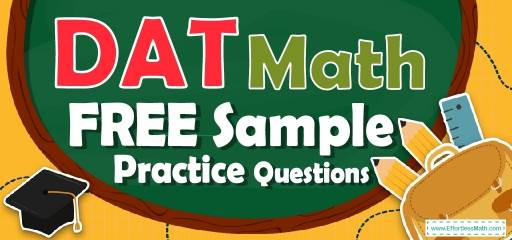# DAT Quantitative Reasoning Math FREE Sample Practice QuestionsPreparing for the DAT Quantitative Reasoning Math test? To do your best on the DAT Quantitative Reasoning Math test, you need to review and practice real DAT Quantitative Reasoning Math questions.  There’s nothing like working on DAT Quantitative Reasoning Math sample questions to hone your math skills and put you more at ease when taking the DAT Quantitative Reasoning Math test. The sample math questions you’ll find here are brief samples designed to give you the insights you need to be as prepared as possible for your DAT Quantitative Reasoning Math test.

Check out our sample DAT Quantitative Reasoning Math practice questions to find out what areas you need to practice more before taking the DAT Quantitative Reasoning Math test!

Start preparing for the 2022 DAT Quantitative Reasoning Math test with our free sample practice questions. Also, make sure to follow some of the related links at the bottom of this post to get a better idea of what kind of mathematics questions you need to practice.

## 10 Sample DAT Quantitative Reasoning Math Practice Questions

1- 6 liters of water are poured into an aquarium that’s 15cm long, 5cm wide, and 60cm high. How many cm will the water level in the aquarium rise due to this added water? (1 liter of water $$=$$ 1000 $$cm^3$$)

A. 80

B. 40

C. 20

D. 10

E. 5

2- If a box contains red and blue balls in the ratio of $$2 : 3$$, how many red balls are there if 90 blue balls are in the box?

A. 90

B. 60

C. 30

D. 10

E. 8

3- If $$|a|<1$$, then which of the following is true? $$(b>0)$$?
I. $$–b<ba <b$$
II.$$-a<a^2<a \space$$ if $$\space a<0$$
III.$$-5<2a-3<-1$$

A. I only

B. II only

C. I and III only

D. III only

E. I, II, and III

4- What is the surface area of the cylinder below?

A. $$48 \space π \space in^2$$

B. $$57 \space π \space in^2$$

C. $$66 \space π \space in^2$$

D. $$288 \space π \space in^2$$

E. $$400 \space π \space in^2$$

5- A chemical solution contains $$4\%$$ alcohol. If there is 24 ml of alcohol, what is the volume of the solution?

A. 240 ml

B. 480 ml

C. 600 ml

D. 1200 ml

E. 2400 ml

6- Which of the following points lies on the line $$2x + 4y = 10$$?

A. $$(2, 1)$$

B. $$(–1, 3)$$

C. $$(–2, 2)$$

D. $$(2, 2)$$

E. $$(2, 8)$$

7- In the following figure, ABCD is a rectangle, and E and F are points on AD and DC, respectively. The area of $$∆$$BED is 16, and the area of $$∆$$BDF is 18. What is the perimeter of the rectangle?

A. 20

B. 22

C. 32

D. 40

E. 44

8- What is the solution of the following inequality?
$$|x-10|≤3$$

A. $$x≥13 ∪ x≤7$$

B.$$7≤x≤13$$

C. $$x≥13$$

D. $$x≤7$$

E. Set of real numbers

9- A bag contains 18 balls: two green, five black, eight blue, a brown, a red, and one white. If 17 balls are removed from the bag at random, what is the probability that a brown ball has been removed?

A. $$\frac{1}{9}$$

B. $$\frac{1}{6}$$

C. $$\frac{16}{17}$$

D. $$\frac{17}{18}$$

E. $$\frac{1}{2}$$

10- If a tree casts a 24–foot shadow at the same time that a 3 feet yardstick casts a 2–foot shadow, what is the height of the tree?

A. 24 ft

B. 28 ft

C. 36 ft

D. 48 ft

E. 52 ft

## Best DAT Quantitative Reasoning Math Prep Resource for 2022

1- A
$$One \space liter=1000 cm^3→ 6 liters=6000 cm3$$
$$6000=15×5×h→h=\frac{6000}{75}=80 cm$$

2- B
$$\frac{2}{3}×90=60$$

3- C
I. $$|a|<1→-10→-ba^2>a$$ (plug in$$-1/2$$, and check!) (It’s false)
III. $$-1<a<1$$,multiply all sides by 2,then: $$-2<2a<2$$
Subtract 3 from all sides. Then:
$$-2-3<2a-3<2-3→-5<2a-3<-1 (It \space is \space true!)$$

4- C
Surface Area of a cylinder $$= 2πr (r + h)$$,
The radius of the cylinder is $$3 (6 ÷ 2)$$ inches and its height is 8 inches. Therefore,
Surface Area of a cylinder $$= 2π (3) (3 + 8) = 66 π$$

5- C
$$4\%$$ of the volume of the solution is alcohol. Let $$x$$ be the volume of the solution.
Then: $$4\%$$ of $$x = 24$$ ml ⇒ $$0.04 x = 24 ⇒ x = 24 ÷ 0.04 = 600$$

6- B
Plug in each pair of numbers in the equation:
A. $$(2, 1): 2 (2) + 4 (1) = 8$$ Nope!
B. $$(–1, 3): 2 (–1) + 4 (3) = 10$$ Bingo!
C. $$(–2, 2): 2 (–2) + 4 (2) = 4$$ Nope!
D. $$(2, 2): 2 (2) + 4 (2) = 12$$ Nope!
E. $$(2, 8): 2 (2) + 4 (8) = 36$$ Nope!

7- D
The area of $$∆$$BED is 16, then:
$$\frac{4×AB}{2}=16→4×$$AB$$=32$$→AB$$=8$$
The area of $$∆$$BDF is 18, then:
$$\frac{3×BC}{2} =18→3×$$BC$$=36$$→BC$$=12$$
The perimeter of the rectangle is $$= 2×(8+12)=40$$

8- B
$$|x-10|≤3→-3≤x-10≤3→-3+10≤x-10+10≤3+10→7≤x≤13$$

9- D
If 17 balls are removed from the bag at random, there will be one ball in the bag. The probability of choosing a brown ball is 1 out of 18. Therefore, the probability of not choosing a brown ball is 17 out of 18 and the probability of having not a brown ball after removing 17 balls is the same.

10- C
Write a proportion and solve for $$x$$.
$$\frac{3}{2}=\frac{x}{24} ⇒ 2x=3 ×24 ⇒ x=36$$ ft

## The Best Books to Ace the DAT Quantitative Reasoning Math Test

### What people say about "DAT Quantitative Reasoning Math FREE Sample Practice Questions - Effortless Math: We Help Students Learn to LOVE Mathematics"?

No one replied yet.

X
23% OFF

Limited time only!

Save Over 23%

SAVE $5 It was$21.99 now it is \$16.99